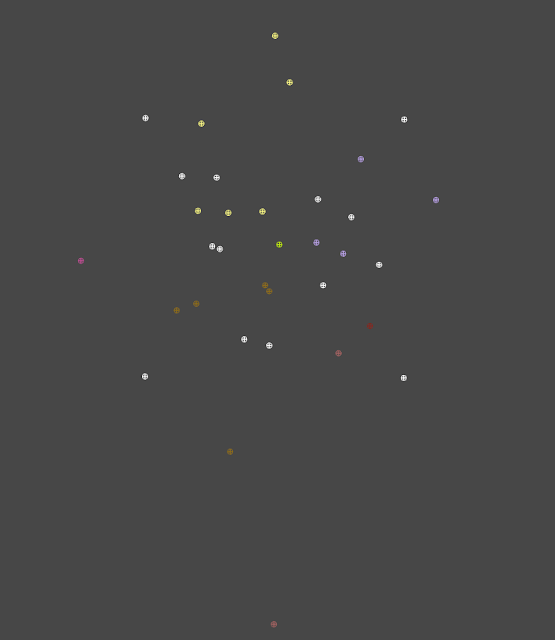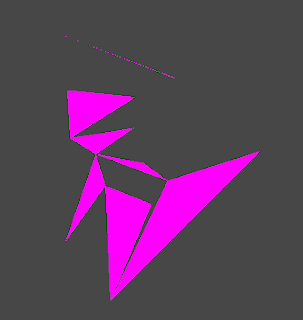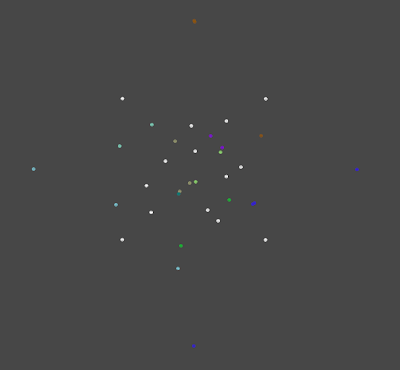### Procedural Geometry from Fracturing

Here’s the follow up to the procedural fracturing post I wrote two weeks ago. As for the assets roadmap I talked about in the last post, I have decided that it doesn’t quite make sense to go over the assets at this point, when so much of that is still up in the air. I’m thinking of creating a dedicated roadmap/status page that gives the current progress on all stuff, for convenient access and updating. Anyways, here’s the juice:

## Why can’t I hold all these vertices?

Last time we went over how to get the vertices out of a voronoi diagram, and I sort of-maybe-not-really fixed the problem I was having earlier with missing some of the vertices. I scrapped the whole compute shader business and switched it over to Unity C# code, and along the way I discovered LINQ (which is like SQL for code?!?!) but that’s another story. Without using a compute shader, it became both easier and harder to debug. Easier because I could now draw gizmos (which are 3D wireframe objects that are only drawn in the scene view) to show where my vertices are in 3D space. And, you know, you can actually use a debugger for C#. Unfortunately, it also removed the ability to see the whole diagram as a texture... so at this point, I don’t really know if I am missing some vertices because I can’t use my cool human mind brain pattern matcher to detect the vertices or to see where they are missing. At any rate, I have some (possibly incomplete) amount of vertices and I would like to turn them into actual triangle meshes for rendering and animation. There are two challenges here: Triangulation, and vertex sorting.Voron gizmos. Voronoi sites are in white, vertices are various colors. Try and see if you can find any missing vertices. (no really, please do).

## TriangulationTerrible garbage (back)
So, we possibly have the vertices for each voron/polygon, and from inspection, some of these vorons have less than 3 vertices (possibly an indication of a bug), so make sure you check that there are at least three vertices for each polygon, because you can’t really draw anything that can’t be turned into triangles. Which brings me to the next fun (terrible) challenge. Maybe you don’t know this, but to draw anything in three-dee graphics you have to have triangles, and not just any triangles- the order of the points in the triangle is important (we’ll get to that later). If you have more than 3 vertices in your polygon, how do you turn that into triangles? There are a few methods for this, some more robust than others, but it’s a confusing topic in general. We can be smart(?) about this and use the knowledge that voronoi diagram sections are guaranteed to be convex to help us a little bit, or maybe we can just copy and paste the ear clipping algorithm located on the Unify wiki. Running the ear clipping algorithm on my raw voron vertices results in this garbage:Terrible garbage (front)

One of the problems here is vertex sorting, the other is that the geometry is actually infinitely thin; we’ll need to sort the vertices to fix the latter.

## Vertex sorting

The direction a triangle ‘faces’ is determined by the winding of the triangle (which also determines the normal direction). If your triangle isn’t facing toward the camera, then it isn’t rendered as long as a common optimization called backface culling is enabled. The winding and normal direction are given by the left hand rule. Literally take your left hand and curl it in the direction of the order of the vertices. Stick your left thumb out, and that is the way the triangle is facing. The problem with the vertices given by a voronoi diagram is that they are given in a completely nonsensical order. Without sorting them, the polygons are invisible or have holes in them.

My first idea was sorting the vertices by starting at the beginning of the vertex list and finding the nearest vertex, and then removing the first vertex, and find the nearest to the next one, and so on. Sorry if that is super hard to follow, but this works because the vorons are guaranteed to be convex. I didn’t spend too much time on this technique because I found out about centroid based sorting soon after.

## Centroid based sorting

After reading this page, I decided to try sorting the vertices in a polygon based on the angle from their centroid. The centroid of a polygon is the average position of all of its vertices: sum(x_positions,y_positions)/number of vertices. You can find the angle between some starting angle (which you’d call zero degrees) and the vector from the centroid to the vertex. The page I linked explains it in decent detail. To get a left handed winding, you’d want to sort the vertices such that the vertices with the highest angle are first. C# has a neato List<T> method called .Sort() which takes a comparison function and sorts a list for you! Sweet! Just write something that compares two vertices based on their centroid angle, and pass it to .Sort(). Done! Well, sort of. I’d post a picture of the sorted vertices, but I actually haven’t finished this part yet, oops.

## Thickening the dimensions

The rest of this post contains my thoughts on how to create an actual 3D volume from the sorted vertices. To get thickness out of these polygons, a simple extrusion would work for me. In Maya, just hit the extrude button on your mesh and you’re done! Oops again, we’re not using Maya, we’re creating vertices at runtime using a pile of half baked algorithms which are held together by hopes and dreams. Ask my friends how many times I have incredulously announced, “I can’t believe this worked!”
The process I am imagining goes like this:
1. Duplicate all vertices and triangles, and offset them by some thickness amount in whatever direction. We’ll call these vertices’ (prime).
2. Add triangles to the triangle list that contain the vertex, vertex’ and one of the adjacent vertices. The next triangle begins with the vertex across from the last vertex you chose. Repeat all the way around the edge until you have a full ring.
3. Cross you fingers.
My probability estimate of this working is 65%. (edit: it actually worked)

That’s it for this week. I’ll post up some pictures of my completed procedural fracturing stuff as soon as I get it working. As always, comments, questions, and requests are appreciated.

## Edit: that was quick

Turns out the algorithm I've been using for finding the circumcircle center was incorrect. With implementing the algorithm on the wikipedia page, things are starting to look a lot less garbage (though still garbage).Even more voron gizmos!